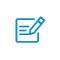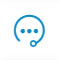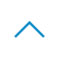# tensorboard 指南

01、名称域
tensorFlow 图表有两种连接关系：数据依赖和控制依赖。数据依赖显示两个操作之间的tensor流程，用实心箭头指示，而控制依赖用点线表示。

02、实现原理
Event: 展示训练过程中的统计数据（最值，均值等）变化情况
Image: 展示训练过程中记录的图像
Audio: 展示训练过程中记录的音频
Histogram: 展示训练过程中记录的数据的分布图

with tf.name_scope('output_act'):
hidden = tf.nn.relu6(tf.matmul(reshape, output_weights) + output_biases)
tf.histogram_summary('output_act', hidden)
Graph的最后要写一句这个，给session回调
merged = tf.merge_all_summaries()

Session 中调用

train_writer = tf.train.SummaryWriter(summary_dir + '/train', session.graph)
valid_writer = tf.train.SummaryWriter(summary_dir + '/valid')

summary, _, l, predictions = session.run([merged, optimizer,loss,train_prediction], options=run_options, feed_dict=feed_dict)

03、借用各个板块的可视化结果来帮助我们调优模型
step 1: 查看 graph 结构
step 2：查看 accuracy，weights，biases
step 3: 修改 code
step 4: 选择最优模型
step 5: 用 embedding 进一步查看 error 出处

04、可视化训练过程代码
tf.histogram_summary()

tf.summary.histogram(layer_name + '/weights', Weights)

tf.r_summary()

tf.summary.scalar('loss', loss)

tf.merge_all_summaries()

sess= tf.Session()

merged = tf.summary.merge_all()

writer = tf.summary.FileWriter("logs/", sess.graph)

sess.run(tf.global_variables_initializer())

for i in range(1000):
sess.run(train_step, feed_dict={xs:x_data, ys:y_data})
if i%50 == 0:
rs = sess.run(merged,feed_dict={xs:x_data,ys:y_data})

tensorboard --logdir logs扫码入群咨询反馈返回顶部